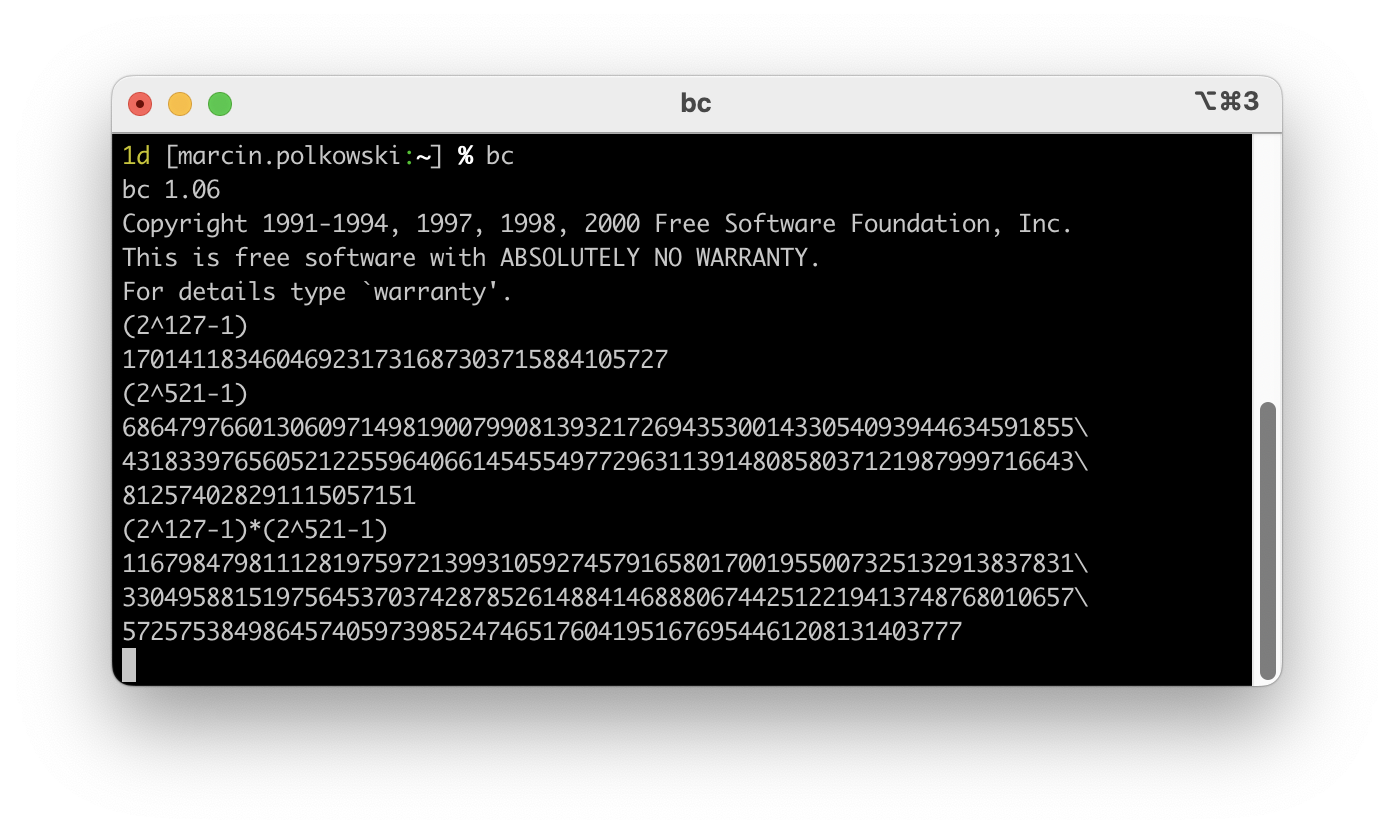## How to calculate the value of the biggest prime we know?

I hope that I don’t need to introduce prime numbers to anyone. They are fascinating for all mathematic enthusiasts. We know a lot of prime numbers, but we have no formula to calculate them. Search for the biggest primes is an ongoing competition. People are looking for the biggest primes among the so-called Mersenne Primes. Mersenne Primes are primes that can be expressed as 2n-1. The record is currently held by 282,589,933−1 with 24,862,048 digits, found by GIMPS in December 2018 (more on Wikipedia).

We can find a value of 282,589,933-1 online (for example beginning and the end of the number on Wikipedia linked above). But what if we would like to calculate the value ourselves? We have multiple options. For the sake of this article, I selected 2:

### bc – an arbitrary precision calculator language

bc is a linux/unix command line calculator that supports arbitrary precision:We can run the following in the terminal to calculate the value of 282,589,933-1. We use tr and sed to pack the result in one line.

``````echo "Testing BC:"
time echo "2^82589933-1" \
| bc \
| tr "\n" " " \
| sed 's/\\ //g' \
> bc.txt
``````

Since the calculation is not trivial the answer is not coming instantaneously. On my 2021 MacBook pro with M1 Max processor it took 30 minutes to get the result: >24 megabytes of digits.

### Python

Yes, Python! Python supports arbitrary precision integers by default. There is a slight complication that was added recently for security reasons: we need to increase the default length of the number that can be converted to a string. Let’s consider the following code:

``````import sys
sys.set_int_max_str_digits(int(3e7))

number = 82589933

print(2**number-1, end=' ')
``````

And run it in the terminal:

``````echo "Testing Python":
time python python.py > python.txt
``````

It takes python almost 3 hours to compute the result.

## Results

Finally, we can confirm that both results are the same:

``````echo "Comparing Results":
shasum bc.txt
shasum python.txt
``````

Which should give result as following:

``````Comparing Results:
9abebcdaf11efd28f1797d6b2744cbcb0cc66e52  bc.txt
9abebcdaf11efd28f1797d6b2744cbcb0cc66e52  python.txt
``````

### Summary

If you ever need to calculate integers bigger then 64 or 128 bits, now you know how.